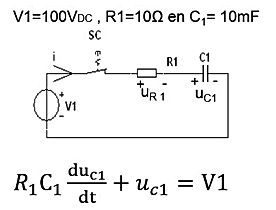naver-site-verification: naver47238a1b6bfbb19a2fd4b619734fa9a6.html
top of page

# Post-processing of the numerical results obtained from the                            differential equation solvers

## Applications of the post-process program Postpre.8xp

This Ti-84 Basic program is a very useful tool for editing the numerical results of the first- and second- order differential solver programs. If we take the transient of the RC circuit as an example, then the solver solves for Uc, but you may also be interested in the current. See the results of Uc below, solved by the differential equation solver (for more information on the first order differential solver, go to:

### The RC circuit analyzed using the Ti-84 first order differential solverThe capacitor voltage Uc is solved by the program. Other variables, such as the current, can be calculated with the post process program. For example, the voltage across the resistor or the circuit current ic.

## The post proces program explainedThe post-processor program calculates from the numerical results :

ady/dx + by + c .

If we want to calculate the current from the calculated capacitor voltage Uc, then it yields that:

ic=C*dUc/dt=10*E-3*dUc/dt (c=10mF=10E-3) therefore a=10E-3, b=0 and c=0.

If we want to calculate the voltage across R1,  then it yields UR1=Usource-Uc=100-Uc and therefore b=-1,c=100

## Numerical Results : capacitor current  and voltage across resistorAbove are the results for Ic en UR1. The calculated current at t=0sec =9.9 A. In reality, the value is 10 A (at t=0sec Uc1=0V en i=100/10=10 A). The calculated value is accurate within 1%, but we have to admit that our step size in the dif. Eq. solver equals.1/100 instead of 0.1/20. At a step size 0.1/20 sec. the calculated current at t=0 sec 9.75 A. An error of 2.5 %.

Conclusion: a smaller step size gives higher accuracy. The error in voltage across the resistance is also within 1 % accuracy.

A better result is obtained by using another formula for Ic, namely Ic=(V1-Uc)/R1, In that case, we don't use the derivative of Uc. For the postprocessor formula a dy/dx + by + c we take a=0, c=100/10=10 and b=-1/10.

(remember y=Uc !!). Finally, we get the following accuracy ( within 0.1%).

A revised version, Postpre2, has been, which gives more possibilities.